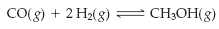×
Log in to StudySoup
Get Full Access to Introductory Chemistry - 5 Edition - Chapter 15 - Problem 52p
Join StudySoup for FREE
Get Full Access to Introductory Chemistry - 5 Edition - Chapter 15 - Problem 52p

Already have an account? Login here
×
Reset your password

# Solved: Consider the reaction. An equilibrium mixture of this reaction at a certainISBN: 9780321910295 34

## Solution for problem 52P Chapter 15

Introductory Chemistry | 5th Edition

• Textbook Solutions
• 2901 Step-by-step solutions solved by professors and subject experts
• Get 24/7 help from StudySoup virtual teaching assistantsIntroductory Chemistry | 5th Edition

4 5 1 414 Reviews
10
3
Problem 52P

Consider the reaction.An equilibrium mixture of this reaction at a certain temperature has [CO] = 0.105 M, [H2] = 0.114 M, and [CH3OH] = 0.185 M. What is the value of the equilibrium constant at this temperature?

Step-by-Step Solution:
Step 1 of 3

Solution: Here, we are going to find the value of the equilibrium constant for the given reaction at the given temperature.

Step1:

The equilibrium constant for a general reaction,

aA + bB ⇄ cC + dD

is expressed as,

Kc = [C]c [D]d / [A]a [B]b

Where [A], [B], [C] and [D] are the equilibrium concentrations of the reactants and products.

Step2:

For the reaction,         CO(g) + 2H2(g) ⇄ CH3OH(g)

Kc = [CH3OH] / [CO] [H2]2

Given,

[CH3OH] = 0.185 M

[CO] = 0.105 M

[H2] = 0.114 M

Substituting the values in the above equation, we get,

Kc = 0.185 / [0.105 x (0.114)2]

= 0.185 / 0.00136458

= 135.57

Thus, the value of the equilibrium constant is 135.57.

------------------------------

Step 2 of 3

Step 3 of 3

##### ISBN: 9780321910295

This full solution covers the following key subjects: temperature, reaction, Equilibrium, mixture, constant. This expansive textbook survival guide covers 19 chapters, and 2046 solutions. The answer to “Consider the reaction. An equilibrium mixture of this reaction at a certain temperature has [CO] = 0.105 M, [H2] = 0.114 M, and [CH3OH] = 0.185 M. What is the value of the equilibrium constant at this temperature?” is broken down into a number of easy to follow steps, and 38 words. Introductory Chemistry was written by and is associated to the ISBN: 9780321910295. This textbook survival guide was created for the textbook: Introductory Chemistry, edition: 5. The full step-by-step solution to problem: 52P from chapter: 15 was answered by , our top Chemistry solution expert on 05/06/17, 06:45PM. Since the solution to 52P from 15 chapter was answered, more than 390 students have viewed the full step-by-step answer.

Unlock Textbook Solution

Enter your email below to unlock your verified solution to:

Solved: Consider the reaction. An equilibrium mixture of this reaction at a certain